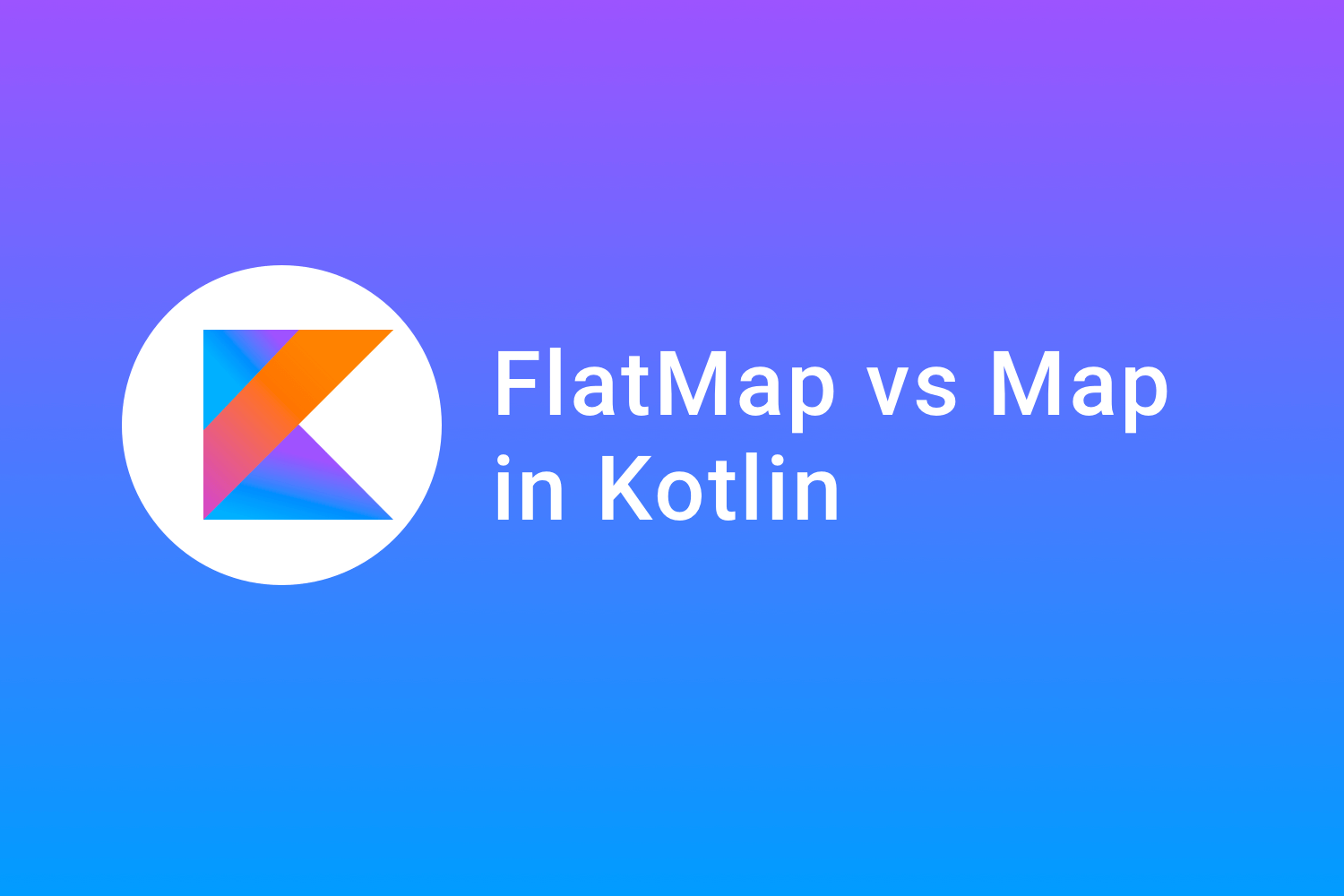# FlatMap vs Map in KotlinWe might have worked with ``` collections ``` in ``` Kotlin ``` while working on our project. In collections, sometime we might have to do some operations to modify it according to our requirements using certain conditions like,

For example, only getting a list of car's names from a list of Cars. Or maybe if we have two lists of cars and bikes then we might need a single list with all the data from the car and bike's list, we would start performing a certain set of iterations to achieve the outcome.

In this blog, we are going to talk about two kinds of ``` transformations ``` that we can use on ``` collections ``` in Kotlin. They are,

• ``` Map ```
• ``` FlatMap ```

#### Map

According to official documentation,

Map Returns a list containing the results of applying the certain set of transformation to each element in the original collection

It basically means that we can modify the list according to our requirements. To understand it better,

Let's say we have a list of integers and we want another list of integers with the elements being the square of the numbers in our original list. Traditionally we can perform these actions using for/forEach loops like,

``````val numbers = listOf(1, 2, 3, 4, 5)
val squaredNumbers = mutableListOf<Int>()

numbers.forEach {
}``````

Here, we first created a ``` mutableList ``` and while ``` iterating ``` in the ``` numbers ``` list we added the ``` square ``` of each item to the ``` squaredNumbers ``` list. And when we print this it will print,

``[1, 4, 9, 16, 25]``

This will print the required list with the square of all numbers.

But, to do this we had to write out 5 lines of code. In Kotlin, we can do this same operation using the map like,

``````val numbers = listOf(1, 2, 3, 4, 5)
val squaredNumbers = numbers.map {
it * it
}``````

Here , we created a list ``` squaredNumbers ``` , and we added items to it from the numbers list by adding some transformation to it.

``` Map ``` helps us to perform a certain transformation to each and every element of the list and it returns a list of newly transformed data. When we print the above ``` squaredNumbers ``` list it will print,

``[1, 4, 9, 16, 25]``

This is the same output as above but with less line of code.

So, basically, the ``` map ``` can be used on collections in ``` Kotlin ``` which will help us to put some transformation to each and every element of the collection and return a new collection.

#### FlatMap

According to official documentation,

Returns a single list of all elements yielded from results of applying certain condition on each element of original collection.

In general terms, ``` FlatMap ``` is used to combine two different collections and returns as a ``` single collection ``` . To understand it better let's consider an example,

let's say we have a data class,

``````data class MotorVehicle(
val name: String,
val model: Int,
val manufacturer: String
)``````

And we have two different collections one of car and other of bikes like,

``````val cars = listOf(
MotorVehicle("Swift", 2016, "Maruti"),
MotorVehicle("Altroz", 2020, "Tata"),
MotorVehicle("Verna", 2019, "Hyundai")
)
val bikes = listOf(
MotorVehicle("R-15", 2018, "Yamaha"),
MotorVehicle("Gixxer", 2017, "Suzuki")
)``````

Both are collections of ``` MotorVehicle ``` and they have a different set of values like one is for car and the other one is for bikes.

What we want to do is, we want to return it as a ``` single list ``` of the type ``` MotorVehicle ``` . The traditional way to do is,

``````val allVehicles = mutableListOf<MotorVehicle>()

This will merge both the collections into one and will print the output.

But we can also perform this using ``` flatMap ``` like let's say we have a collection of both the lists like,

``val vehicles = listOf(cars, bikes)``

Here, vehicles are a list of lists of cars and bikes and we want to merge all the elements from cars and bikes to one list.

We can do it like,

``val allVehicles = vehicles.flatMap { it }``

and when we print the above code, it will print the following,

``[MotorVehicle(name=Swift, model=2016, manufacturer=Maruti), MotorVehicle(name=Altroz, model=2020, manufacturer=Tata), MotorVehicle(name=Verna, model=2019, manufacturer=Hyundai), MotorVehicle(name=R-15, model=2018, manufacturer=Yamaha), MotorVehicle(name=Gixxer, model=2017, manufacturer=Suzuki)]``

Here, ``` flatMap ``` here took each and every item from the list and then combined it into one list.

#### Using Map and FlatMap together.

Let' s say we want a list of only manufacturers from the list,

``val vehicles = listOf(cars, bikes)``

We can get the output by using both the ``` map ``` and ``` flatMap ``` ``` transformations ``` together. So, to get the output we will use,

``````val manufacturerList = vehicles.flatMap {
it
}.map {
it.manufacturer
}``````

Here, what we did was first we merged all the items of ``` vehicles ``` into one list using ``` flatMap ``` and then we mapped out only the manufactures from the combined list using ``` map ``` transformation.

It will print,

``[Maruti, Tata, Hyundai, Yamaha, Suzuki]``

Here, it printed the list of manufacturers which is of type string.

#### Conclusion:

• ``` FlatMap ``` is used to combine all the items of lists into one list.
• ``` Map ``` is used to transform a list based on certain conditions.

Happy learning.

Team MindOrks :)